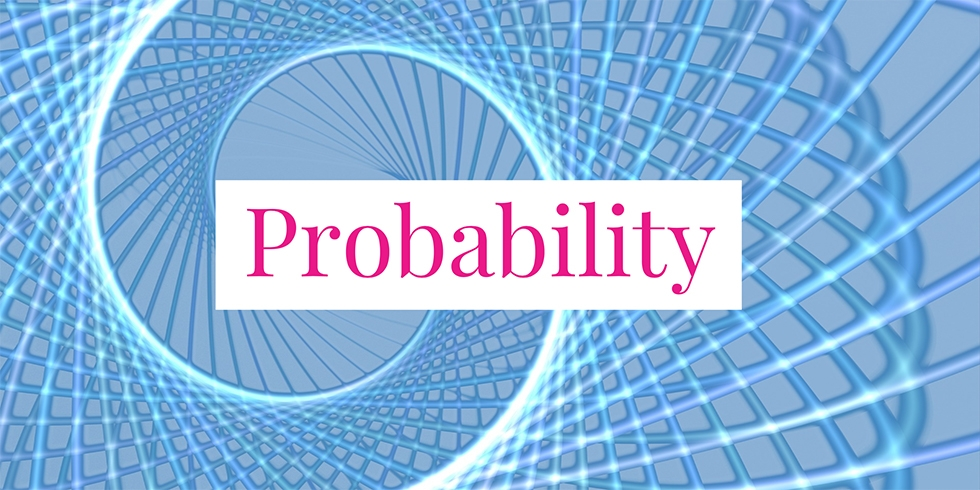# What is the Probability that All Coins Land Heads When Four Coins are Tossed If…?## Problem 730

Four fair coins are tossed.

(1) What is the probability that all coins land heads?

(2) What is the probability that all coins land heads if the first coin is heads?

(3) What is the probability that all coins land heads if at least one coin lands heads?Add to solve later

## Solution.

### Solution (1)

There are $2^4=16$ total possible outcomes of which only one outcome gives rise to all heads.

Thus the probability that all coins land heads is $1/16$.

### Solution (2)

Consider the event that the first coin is heads.

In this case, there are total $2^3=8$ possible outcomes for the rest of coins (2nd, 3rd, and 4th).

Hence, the probability that all coins land heads given that the first coin is heads is $1/8$.

### Solution (3)

Let $H$ be the event that all coins land heads. Let $F$ be the event that at least one coin lands heads. Then the required conditional probability is given by
\begin{align*}
P(H \mid F) &= \frac{P(H \cap F)}{P(F)}.
\end{align*}
The complement $F^c$ of $F$ is the event that all lands tails whose probability $P(F^c)$ is $1/16$ just like part (a). Hence
$P(F) = 1 – P(F^c) = 1 – \frac{1}{16} = \frac{15}{16}.$

It follows that
\begin{align*}
P(H \mid F) &= \frac{P(H \cap F)}{P(F)}\\[6pt] &= \frac{P(H)}{P(F)}\\[6pt] &= \frac{1/16}{15/16}\\[6pt] &= \frac{1}{15}.
\end{align*}Add to solve later

### More from my site

#### You may also like...

This site uses Akismet to reduce spam. Learn how your comment data is processed.

###### More in Probability##### Pick Two Balls from a Box, What is the Probability Both are Red?

There are three blue balls and two red balls in a box. When we randomly pick two balls out of...

Close